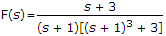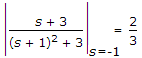# Electronics and Communication Engineering - Signals and Systems - Discussion

47.

If, the coefficient of term e-t in f(t) will be

 [A]. 1 [B]. 0 [C]. 0.5 [D]. 2/3

Explanation:

Coefficient of e-t =.

 Shifaat Rehman said: (Aug 4, 2018) Why only square the term (s + 1)? Why not cube?